# Elementary fuzzy logic glossary

• fuzzy set : A fuzzy set is defined by its membership function. A point in the universe,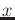, belongs to a fuzzy set,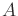with a membership degree,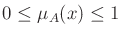.

Figure 10 shows a triangle membership function.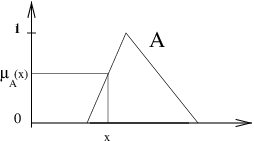• Fuzzy set prototype: a point is a fuzzy set prototype if its membership degree is equal to 1.

• Operators:
•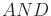: conjunction operator, denoted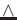, the most common operators are minimum and product.

•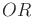: disjunction operator, the most common are maximum and sum.

•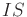: the relation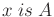is quantified by the membership degree ofin the fuzzy set.

• Partitioning: Partitioning is the definition of the fuzzy sets for a variable definition range. These sets are denoted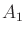,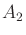, ...

• Standardized fuzzy partition: a fuzzy partition of the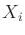variable is called a standardized fuzzy partition if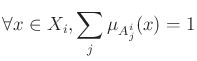.

• Item : an item or individual is composed of a p-dimensional input vector, and eventually of a q-dimensional output vector.

• Fuzzy rule: A fuzzy rule is written as If situation Then conclusion. The situation, called rule premise or antecedent, is defined as a combination of relations such asfor each component of the input vector. The conclusion part is called consequency or conclusion.

• There are two main types of fuzzy rules:

1. Mamdani type. The rule conclusion is a fuzzy set.
The rule is written as: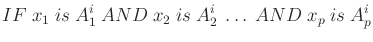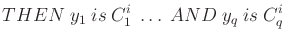where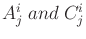are fuzzy sets defining the iput and output space partitioning.

2. Takagi-Sugeno type. The rule conclusion is a crisp value.
The conclusion of the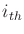rule for the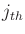output is calculated as a linear function of the input values: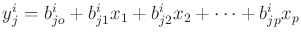, also denoted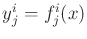.

• Uncomplete rule: A fuzzy rule is said to be uncomplete if its premise is defined by a subset of the input variables. For instance, the rule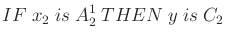is an uncomplete rule, as the variable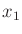does not appear in its premise. Expert rules are generally uncomplete rules. Formally an uncomplete rule can be rewritten as an implicit combination of logical connectorsandoperating on all the variables. If the universe of the variableis split into three fuzzy sets, the above rule can also be written as: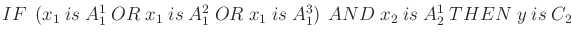.

• Matching degree: For a given data item and a given rule, the rule matching degree, or weight, is denoted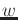. It is obtained by the conjunction of the premise elements: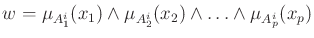, where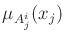is the membership degree of the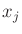value in the fuzzy set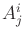.

• Activation: An item is said to activate a rule, if the rule matching degree for the item is greater than zero.

• Rule prototype: an item is a rule prototype if the rule matching degree for this item is equal to 1.

• Fuzzy inference system (FIS): A fuzzy inference system is composed of three blocks, as shown in Figure 11. The first block is the fuzzification block. It transforms numerical values into membership degrees in the different fuzzy sets of the partition. The second block is the inference engine, with the rule base. The third one implements the defuzzification stage if necessary. It yields a crisp value from the rule aggregation result. The number of rules in the FIS is denoted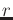.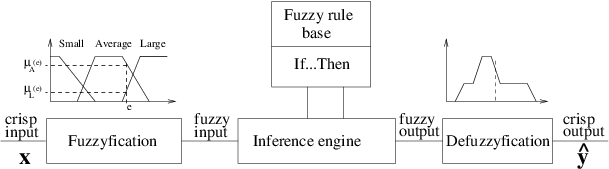• System inferred output: denoted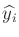for theitem. The inferred value, for a given input, depends both on the rule aggregation and the defuzzification operators.

Rule aggregation is done in a disjunctive way, meaning that each rule opens a possible range for the output. The two main operators are the maximun and the sum. The resulting levels are,being the number of rules and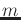the number of labels in the output partition:

• max: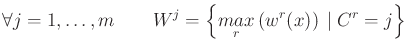• sum: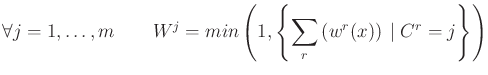Several defuzzification operators are available. Figure 12 illustrates the process, when two labels have a non null resulting level, for two main kinds of operators.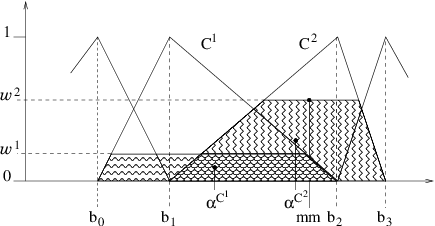The inferred system output for the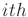example is noted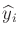.

The mean of maxima operator yields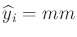. This operator only considers the segment defined by the maximum level. It mainly works within a single linguistic label. Others similar outputs are possible, for example the minimum value of the maximum level or the maximum one.

The weighted area technique favors interpolation between linguistic terms. The output is equal to: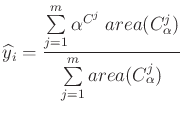(1)

whereis the number of fuzzy sets in the partition,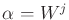is the resulting level of the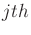fuzzy set,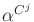is the x-coordinate of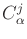centroid, andis a new fuzzy set, defined fromas: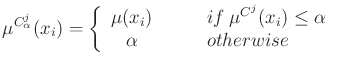• Supervised learning: It induces an input-output mapping from a data set, called learning data set. It is usually limited to a MISO (multiple input single output) system. The learning data set includes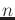items.

• Mean square error: denoted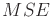, it is equal to: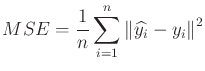• Mean error: Contrary to the previous one, it is homogeneous to a data item. It is expressed as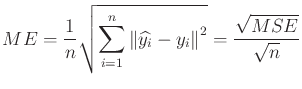(2)